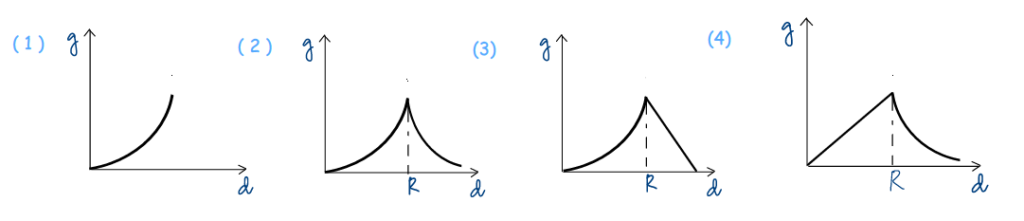# SOLVE JEE (MAIN) 2017 WITH A SMILE – III

1. Home
2. /
3. /
4. SOLVE JEE (MAIN) 2017 WITH A SMILE – III

### Let Us YOU Solve Physics JEE (MAIN) 2017 Physics Paper

We encourage students to attempt more Physics MCQs from JEE (Main) 2017, Paper-1. As usual, our job is limited to linking a question to relevant theory(ies), and no further. You can study the theories from a large pool of articulate, lively video lectures @ PhysicsAcademyOnline.com.. As you solve the questions on your own, expertise and confidence build up bit by bit in you, making you ready for the Engineering and Medical Entrance Examinations. A video solution at the end of every question lets you know, eventually, if you got the correct answer.

Question 07:   The variation of acceleration due to gravity, g, with distance, d, from the centre of the earth is best represented by (R is earth’s radius):The shape of the g vs d graph depends on how the acceleration due to gravity, g, varies below and above earth’s surface. How does g vary with depth below the ground? Subscribe to www.PhysicsAcademyOnline.com, and find the relevant formula here:   Chapter Name – Mechanics; Category –  Basic; Topic Name – Gravitation; Video Name – Variation of Acceleration Due to Gravity I.

The shape of the left part of the graph for d ≤ R can be predicted. How does g vary with altitude above the ground? The relevant formula is derived in the same lecture mentioned above .

The shape of the right part of the graph for d ≥ R can be predicted. Are you still waiting to pick your choice?

I know it, I know it. Smart students have stopped following me after the first step! Because they noticed only one option gives the correct shape of graph for d ≤ R. So why should they waste more time with this question? MCQs sometimes offer such pleasant shortcut.

As a matter of fact, our lectures @ PhysicsAcademyOnline.com. are so exhaustive, you will find the same graph within the above lecture .

Question 08:  A copper ball of mass 100 g is at a temperature T. It is dropped in a copper calorimeter of mass 100 g, filled with 170 g of water at room temperature 30°C. Subsequently, the temperature of the system is found to be 75°C. Specific heat of copper is 0.1 cal/g-°C. T is:

(1) 800°C                  (2) 885°C                    (3) 1250°C                         (4) 825°C

After the ball is dropped, on what principle does heat exchange take place between different agents? Study from the lecture: Chapter Name – Heat and Thermodynamics; Category –  Basic; Topic Name – Calorimetry and Phase Change; Video Name – Principle of Calorimetry, and Determination of Specific Heat by Method of Mixtures.

How much heat is lost, and by which agent(s)? How much heat is gained, and by which agent(s)? Although the above lecture discusses a similar situation, to learn from the basics follow:   Chapter Name – Heat and Thermodynamics; Category –  Basic; Topic Name – Calorimetry and Phase Change; Video Name – Definition of Heat and Types of Heat Capacity.

A simple calculation gives T.